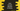# Java Program to convert Decimal to Binary in 3 ways## Java program to convert Decimal to Binary Number :

In this tutorial, we will check how to convert a decimal number to binary. We will use three different methods to convert one decimal number to its binary form.

### Decimal :

For denoting integer and non-integer numbers, decimal number system uses 10 different digits, 0,1,2,3,4,5,6,7,8 and 9 i.e it is a base 10 number system. Also known as the Hindu-Arabic numeral system, decimal is the mostly used numeral system.

### Binary :

The binary numeral system represents numeric values using 0 and 1. It is a base 2 system and each digit is known as ‘bit’.

Let’s take a look into the code :

## Method 1: Using an array of int :

In this method, we will use one array to store all reminders. And finally iterating through the array, we will print it out :

``````static void convertToBinary(int no){
int[] container = new int;

int i = 0;

while (no > 0){
container[i] = no%2;
i++;
no = no/2;
}

for (int j = i -1 ; j >= 0 ; j--){
System.out.print(container[j]);
}
}
``````

## Method 2: Using StringBuilder :

This method is similar to the previous one, but instead of using an array we will use one StringBuilder and append each reminder :

``````static void convertToBinaryUsingString(int no){
StringBuilder result = new StringBuilder();

int i =0;

while (no > 0){
result.append(no%2);
i++;
no = no/2;
}
System.out.println(result.reverse());

}
``````

## Method 3: Using inbuilt method public static String toBinaryString(int i) :

If you don’t want to find out the binary representation in one line, then “Integer” class has one static method known as toBinaryString(int). Use this method directly :

``````System.out.print(Integer.toBinaryString(no));
``````

### Full class :

``````import java.util.Scanner;

public class Example {

public static void main(String[] args) {
System.out.println("Please enter the number you want to convert : ");
Scanner scanner = new Scanner(System.in);

int no = scanner.nextInt();

if (no > 0) {
System.out.println("Binary conversion for " + no + " is :");

System.out.println("Using Array :");
convertToBinary(no);

System.out.println("\nUsing StringBuilder :");
convertToBinaryUsingString(no);

System.out.println("\nUsing toBinaryString() :");
System.out.print(Integer.toBinaryString(no));

}

}

static void convertToBinary(int no) {
int[] container = new int;

int i = 0;

while (no > 0) {
container[i] = no % 2;
i++;
no = no / 2;
}

for (int j = i - 1; j >= 0; j--) {
System.out.print(container[j]);
}
}

static void convertToBinaryUsingString(int no) {
StringBuilder result = new StringBuilder();

int i = 0;

while (no > 0) {
result.append(no % 2);
i++;
no = no / 2;
}
System.out.println(result.reverse());

}
}``````

If you run this, it will give output as like below:

``````Please enter the number you want to convert :
12
Binary conversion for 12is :
Using Array :
1100
Using StringBuilder :
1100

Using toBinaryString() :
1100``````